Next: About this document ... Up: lab_template Previous: lab_template

Subsections

# Polar Coordinates

## Background

The use of polar coordinates allows for the analysis of families of curves difficult to handle through rectangular coordinates. If a curve is a rectangular coordinate graph of a function, it cannot have any loops since, for a given
value there can be at most one correspondingvalue. However, using polar coordinates, curves with loops can appear as graphs of functions

## Plotting Polar Curves

When you graph curves in polar coordinates, you are really working with parametric curves. The basic idea is that you want to plot a set of points by giving their coordinates in
pairs. When you use polar coordinates, you are defining the pointsin terms of polar coordinates
. When you plot polar curves, you are usually assuming that
is a function of the angle
andis the parameter that describes the curve. In Maple you have to put square brackets around the curve and add the specification coords=polar. Maple assumes that the first coordinate in the parametric plot is the radiusand the second coordinates is the angle
.

This example is a cardiod.

>plot([1-cos(theta),theta,theta=0..2*Pi],coords=polar,view=[-2..2,-2..2]);

It is not always apparent what domain you need to get the full curve. You can use ParamPlot to animate the plot.
>with(CalcP7):
>ParamPlot([1-cos(theta),theta],theta=0..Pi,coords=polar);

To begin the animation, click on the plot and a new tool bar appears where the buttons resemble vcr controls. Click on the go-arrow. You didn't get the full curve, change the domain until you do.

## Area in Polar Coordinates

The relationship between area and integrals in polar coordinates is a little strange; the area inside a circle given (in polar coordinates) by
is NOT just
. Here is the rule: Area inside
is given by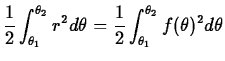. This comes from the fact that the area in a thin wedge with radiusand angleis
. Note that this gives you the right answer for a circle:. So to find the area of the cardiod use the following command.
>Area1:=1/2*int((1-cos(theta))^2, theta=0..2*Pi);
>evalf(Area1);


## Exercises

Note: Keep your work well organized and clearly labeled. The equation of a rose is
. For six consecutive integer values of
do the following four items.
1. Plot the rose using ParamPlot and find the domain necessary to trace the entire curve without retracing any part of it.
2. For whatvalues will begin and end the trace of one petal. (Use the ParamPlot or use what you know about when cosine equals zero).
3. Using plot, plot one petal.
4. Find the area of one petal and then find the area of the entire rose by multiplying or by changing the domain.

A final question, how is the value ofrelated to the number of petals of the rose?Next: About this document ... Up: lab_template Previous: lab_template
Jane E Bouchard
2004-09-29